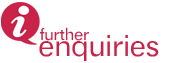﻿﻿﻿﻿﻿﻿﻿ Research Programme | INSTITUTE FOR MATHEMATICAL RESEARCH
» LABORATORIES » Laboratory of Computational Statistics and Operations Research » Research Programme

# Research Programme

1. Modelling and Computational Statistics

This programme consists of time series, spatial modeling, robust statistics, bootstrapping, extreme value theory, environmetrics, survival analysis, medical statistics and biostatistics. Currently, research is focused on Generalised Time Series Models. Spatial modelling involves modelling data observed on a two dimensional plane based on the observed spatial autocorrelation structure. Robust statistics is useful for inference when classical statistical measures and inference fails. Extreme value theory is appropriate when handling extreme data and predicting extreme events. The field of extreme value theory is currently blended with other fields such as spatial modeling, Bayesian modeling and financial modeling. Currently, research is undertaken in spatial extreme modeling and environmental modeling. Survival analysis deals with death in biological organism and failure in mechanical systems. Medical statistics deals with applications of statistics in health and medicine. Biostatistics is the application of statistics in biology and agriculture. Besides knowledge enhancement, the research conducted in this programme is useful for industries like MPOB and NAHRIM with whom linkages have been established.

2. Computational Operations Research

The field of Computational Operations Research provides a platform for the application of computers and operations research techniques to problems in many scientific fields, among others, engineering, medical, transportation, economics, inventory control, safety, and urban planning. The common element in all the scientific areas that this field addresses is the need for some optimization methodology for determining viable solutions to problems, using computers and the techniques of operations research. However, it is not only the methodology which is of interest, the applications are of equal importance. The two are mutually supportive, since understanding the application helps one greatly to comprehend the optimization methods used, and vice versa. This field comprises of the following sub-fields: mathematical programming, optimization, optimal control, and operations research.

A brief description of these sub-fields are as follows:

• Mathematical Programming is the use of mathematical models, particularly optimizing models, to assist in making decisions. The models will include a number of variables to represent the decisions to be made, and a number of inequalities (or equations) to represent the constraints on the decisions. In broad terms, mathematical programming can be defined as a mathematical representation aimed at programming or planning the best possible allocation of scarce resources. Its particular characteristic is that thebest solution to a model is found automatically by optimization software.
• Optimizationis the selection of a best element (with regard to some criterion) from some set of available alternatives. In the simplest case, an optimization problem consists of maximizing or minimizing a real function by systematically choosing input values from within an allowed set and computing the value of the function. More generally, optimization includes finding "best available" values of some objective function given a defined domain (or input), including a variety of different types of objective functions and different types of domains.
• Optimal control theory, an extension of the calculus of variations, is a mathematical optimization method for deriving control policies. Optimal control deals with the problem of finding a control law for a given system such that a certain optimality criterion is achieved. An optimal control is a set of differential equations describing the paths of the control variables that minimize the cost function. The optimal control can be derived using Pontryagin's maximum (or minimum) principle (a necessary condition) or by solving the Hamilton–Jacobi–Bellman equation (a sufficient condition).
• Operations Research is a scientific approach to complex decision making that seeks to best design and operate a system, usually under conditions requiring the allocation of scarce resources. The discipline of operations research is distinguished by its emphasis on modelling real world systems and analysing their behaviour using a variety of mathematical and computational techniques such as data envelopment analysis, fuzzy theory, heuristics and metaheuristics. Sample applications include machine scheduling, cutting and packing, routing and transportation, supply chain management, timetabling, and production planning.

Updated:: 11/04/2017 [iszuanie]

MEDIA SHARING